# The final project of CS348B An Alternative to the Best-Candidate Sampling Patterns - Xiaohu Zhang (Tom) -

Project Goal

I changed my theme of the final project. My proposal was to render thin film interference, e.g. soap bubbles. Click here to see my original proposal. But what I do for the final project is an alternative to the best-candidate sampling algorithm.

Basic Idea

The best-candidate algorithm is an approach to generate sampling patterns mimic the Poisson disk patterns. It has one advantage over the dart throwing algorithm in that any prefix of the final pattern is well distributed.

The best-candidate algorithm works like this. It adds points to the pattern one by one. For every new point, the algorithm generates a large number of candidates and then picks the one which is farthest away from all old samples. So here comes my alternative. Why don't we just pick the one point in the square (or torus) which is indeed the farthest away from all old points? Then the algorithm turns to a deterministic one. The study of the pros and cons of deterministic algorithms compared to their randomized versions is very complicated and will not be addressed here.

There are two approaches for computing the furthest site. The first one is an approximate algorithm which only considers points on a fine grid, and will use brutal force to compute the distances. The second one is based on the Delaunay triangulation.

Brutal Force Approach

This approach is basically a variation of the best-candidate algorithm. The difference is that we consider a huge number of fixed candidates here, instead of a moderately large number of random candidates. The simplest choice for these candidates is a grid, for example, a 1000*1000 grid, or 10000*10000 grid. There're other more efficient alternatives, for example, hexagonal grid, 2-d Latin Hypercube samples, or other low discrepancy point set. But here I only implemented the simplest grid.

Initialization We need to supply at least two initial points to get a good sampling pattern. If we have just one initial point, then the result will have a grid structure, (remember that we use the torus here.) We use a matrix (same size as the grid) to store the minimum distances from each candidate point to existing points in our sampling pattern. Once we have the initial points, we will initialize the distance matrix. This step costs a lot of computation!

Loop Then we look for the biggest entry in this matrix. The corresponding point will be added to the sample pattern. We then update the distance matrix and find the next point. We continue this loop until we find the desired number of points.

A little bit optimization When we do updating of the distance matrix, we only need to consider the candidates near the newest point "P" in our sampling pattern. In fact, let's denote the minimum distance of the newest point to all the older points as "d", and draw a circle around "P" with radius "d", then we only need to consider the candidates falling inside this circle. To make things easier, we consider a square (centered at "P", side length 2*"d") instead of the circle.

If we have more than a few initial points, we can do it more clever. The idea is like the above. We loop over the initial points, and update their neighborhood (the size should be computed correctly.)

Complexity analysis Suppose our grid has N candidates and we need M points in our final point set. My experiment has N = 1 million, M = 4096.

space We need to keep the distance matrix. We can store this matrix as an interger matrix, (similar to the best-candidate algorithm in lrt, we use the square of Euclidean distance to avoid unnecessary square root computation). The space complexity is O(N). More detailed, if we use 1000*1000 grid, we need 4M memory; if we use 10000*10000 grid, we need 400M memory.

time Finding the biggest entry in the matrix M times takes time O(MN). For the i-th point, updating the distance matrix takes time C*N/i. This is because that the size of the neighborhood is inversely proportional to i. So updating the matrix will take time O(Nlog(M)). However, based on my experience, the time to find the biggest entry is neglectable compared to the time to update the distance matrix, when M is much smaller than N, which is of most the cases.

Postprocessing In fact, 1000*1000 grid is not fine enough for several thousands of points, so I think we can do jittering for the final result. The jitter amount should be controlled under half of the grid smallest spacing.

Delaunay Triangulation Approach

In fact, the standard way to compute furthest site is to do Delaunay triangulation. This is in fact a much better way than the brutal force approach. I won't talk about this too much because this is not a very new idea.

This algorithm can be very fast. Since the points are well distributed, updating the triangulation costs little time. One thing we need to take extra care is points near the boundary. There're two approaches to deal with this. First, we extend the square to a torus, and add copies of points explicitly outside of the square. Second, we can generate points in a larger area, and crop the region to get what we want.

The deterministic nature of this approach might make a lot of people unhappy. There're two simple ways to inject some randomness. The first is like the folowing, when we are adding a new point, we don't have to add the circumcenter of the triangle with the biggest circumradius, we can consider those triangles with the second, third, fourth, ... biggest circumradii, and give their circumcenters some probability to enter the sampling set. For the second method, we can give a small random jitter to each newly admitted point, and this jitter amount decreases as more and more points are getting into the point set. Both methods are promising I think.

Generated point sets
Click on each picture to see the full size version

Generated by the brutal force approach

Jittering the pattern generated by the brutal force approach

Generated by the Delaunay triangulation approach

Generated by the best-candidate algorithm

The points generated by the brutal force don't seem to cover the square as uniformly as the other two methods. I think this is because the grid is not fine enough. I guess 10000*10000 will be sufficient for 4096 points.

Comparing these point sets

Here we render a simple scene of checkerboard, to see the aliasing effect of these sampling patterns. Here we will compare the point sets generated by our algorithms and those generated by the three samplers provided by lrt. The scene is rendered using the perspective camera, so it only uses the 2-d image samples, not the time samples and lens samples. There're five algorithms being compared below: the brutal force (jittered), the Delaunay triangulation, the best-candidate, the stratified, the low discrepancy.

The pictures on the left were rendered with "PixelSamples 1 1" (under-sampled) and the pictures on the right were rendered with "PixelSamples 2 2". The aliasing effect is most significant in the picture rendered by the low-discrepancy sample pattern. And the two new algorithms generate similar quality pictures as the best-candidate sampling pattern does.

 PixelSamples 1 1 PixelSamples 2 2 Brutal force (jittered)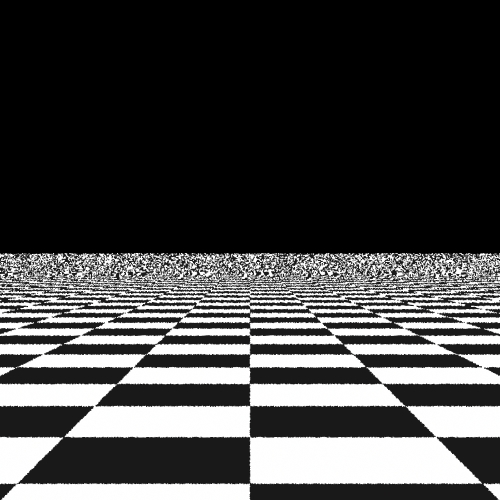Brutal force (jittered)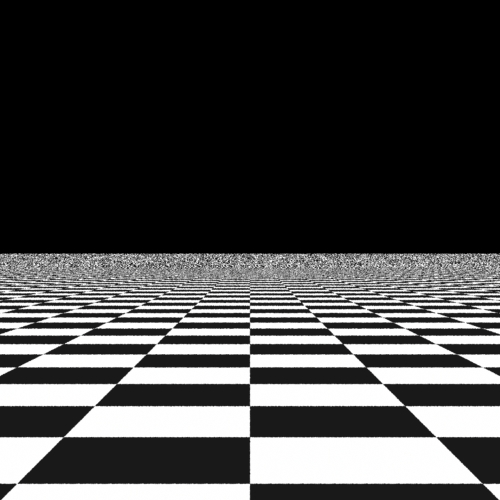Delaunay triangulation approach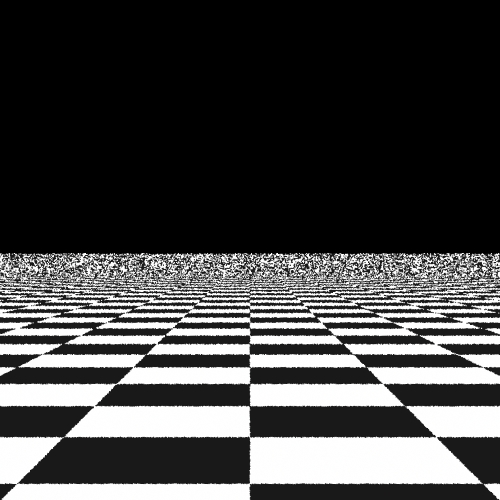Delaunay triangulation approach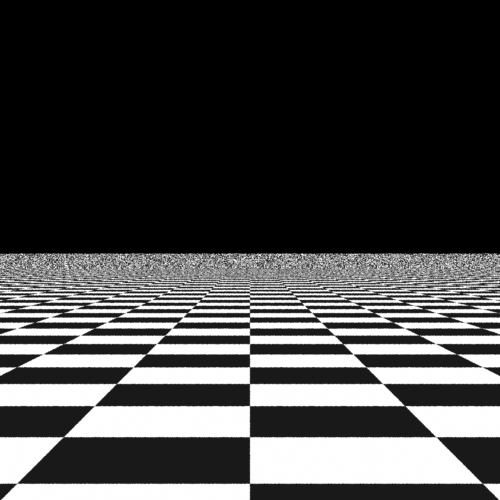Best-candidateBest-candidateStratified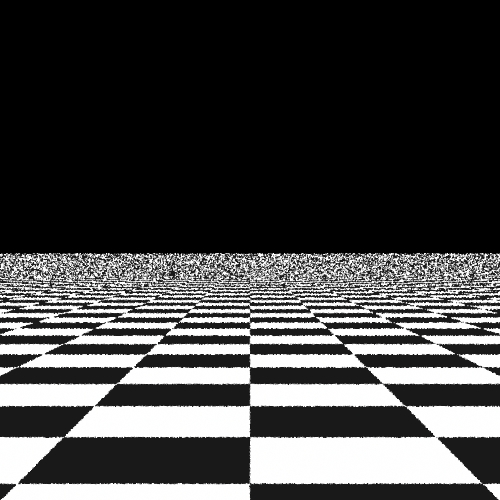Stratified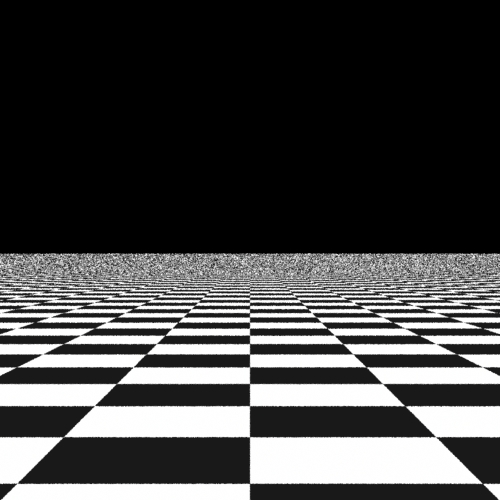Low discrepancy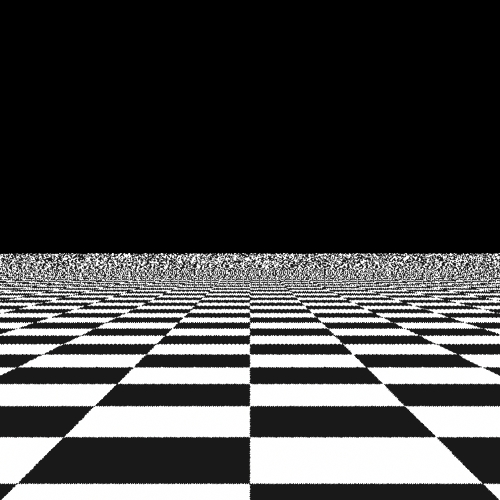Low discrepancyRandom (IID)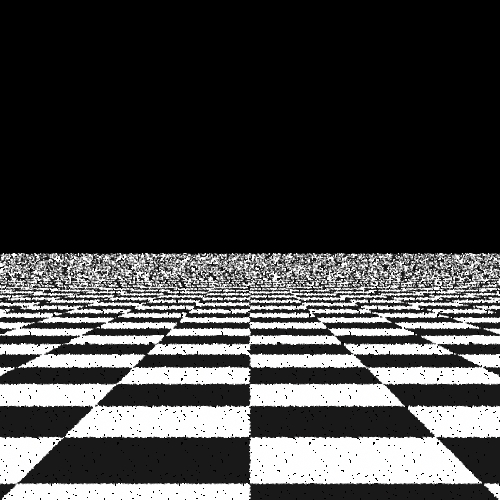Random (IID)Conclusions

I didn't render soap bubbles. Soap bubbles are wonderful but I failed to render them, miserably. All I could get is the color bands people see in their physics textbooks. They don't appear to be like soap bubbles at all. I spent a lot of time on researching the physics of thin film interference, colorimetry, bubble/foam geometry, previous cs348b work, but I couldn't get theory turn into working codes.

Instead, I came up with an alternative to the best-candidate algorithm implemented in lrt and wrote two algorithms to realize it (in Matlab). I generated point sets using these algorithms. To make the comparison, I used UltraEdit to insert my point set to the file "sampledata.cc" and manually remade the "bestcandidate.so". This was awkward, I admit. But I didn't have a lot of time after I made up my mind to give up the soap bubble. And I have lost confidence on C coding too.

To generate 4096 points, the brutal force algorithm takes 38 minutes, and the Delaunay triangulation algorithm takes 15 minutes. I haven't optimized the second algorithm to the best. I expect the optimized version will take only about one minute, thus it's quite promising. I intended to do a comparison of time cost, but I couldn't get the "samplepat" run. So I don't know how much time the best-candidate algorithm will use.